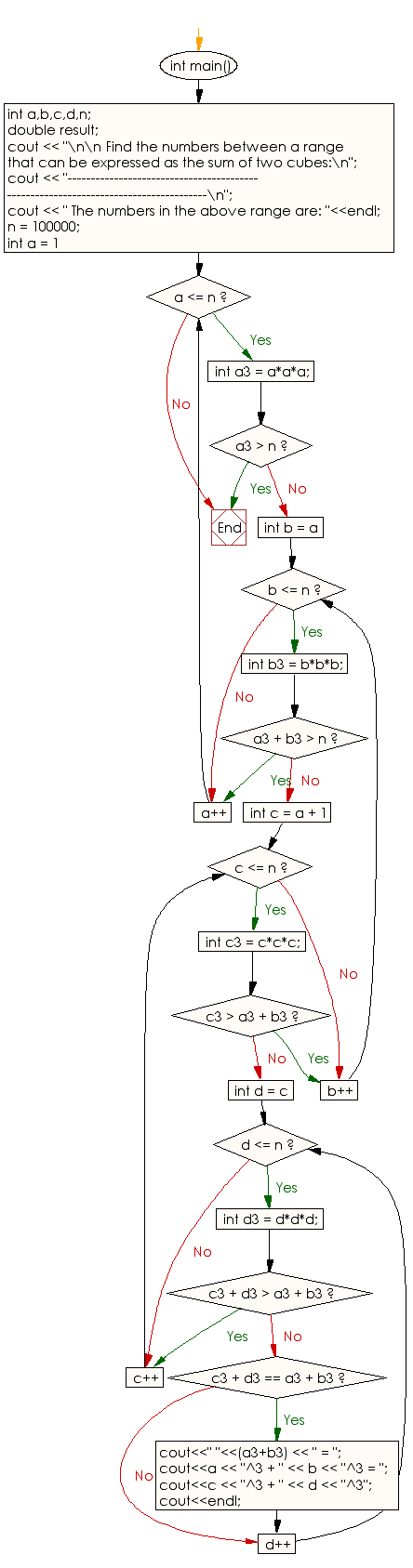﻿ C++ : Sum of two cubes between 1 and n in two different ways

# C++ Exercises: Find any number between 1 and n that can be expressed as the sum of two cubes in two different ways

## C++ Numbers: Exercise-34 with Solution

Write a program in C++ to find any number between 1 and n that can be expressed as the sum of two cubes in two (or more) different ways.

Sample Solution:

C++ Code :

``````# include <iostream>
# include <math.h>
using namespace std;
int main()
{
int a,b,c,d,n;
double result;
cout << "\n\n Find the numbers between a range that can be expressed as the sum of two cubes:\n";
cout << "------------------------------------------------------------------------------------\n";
cout << " The numbers in the above range are: "<<endl;
n = 100000;
for (int a = 1; a <= n; a++)
{
int a3 = a*a*a;
if (a3 > n) break;

for (int b = a; b <= n; b++)
{
int b3 = b*b*b;
if (a3 + b3 > n) break;

for (int c = a + 1; c <= n; c++)
{
int c3 = c*c*c;
if (c3 > a3 + b3) break;

for (int d = c; d <= n; d++)
{
int d3 = d*d*d;
if (c3 + d3 > a3 + b3) break;

if (c3 + d3 == a3 + b3)
{
cout<<" "<<(a3+b3) << " = ";
cout<<a << "^3 + " << b << "^3 = ";
cout<<c << "^3 + " << d << "^3";
cout<<endl;
}
}
}
}
}
}
``````

Sample Output:

```Find the numbers between a range that can be expressed as the sum of two cubes:
------------------------------------------------------------------------------------
The numbers in the above range are:
1729 = 1^3 + 12^3 = 9^3 + 10^3
4104 = 2^3 + 16^3 = 9^3 + 15^3
13832 = 2^3 + 24^3 = 18^3 + 20^3
39312 = 2^3 + 34^3 = 15^3 + 33^3
46683 = 3^3 + 36^3 = 27^3 + 30^3
32832 = 4^3 + 32^3 = 18^3 + 30^3
40033 = 9^3 + 34^3 = 16^3 + 33^3
20683 = 10^3 + 27^3 = 19^3 + 24^3
65728 = 12^3 + 40^3 = 31^3 + 33^3
64232 = 17^3 + 39^3 = 26^3 + 36^3
```

Flowchart:C++ Code Editor: